# Deep dive on how to write DataWeave curried functions

## Overview

In DataWeave, you can dynamically solve problems with different approaches due to the functional programming features of the language. Currying is a function that takes multiple arguments into numerous functions with just a single argument. For example, a normal function will take two arguments such as multiply(x,y). However, when a function is curried, it can only take one argument at a time multiply(x)(y). The value x and y can be fixed, which means the body of the function is executed and the result is returned.

Currying helps you avoid passing the same variable again and again. If x is fixed then the returning function can be reused multiple times and x will not need to be fixed again on that function. Currying can help you create a higher-order function. The curried function takes a function as an argument and returns a function, thus increasing the opportunity to reuse the function in other parts of your DataWeave script.

If you are new to MuleSoft, don’t forget to click the button below to signup for a free trial and check out our MuleSoft Fundamentals Course if you are looking for a good place to get started.

## Simple DataWeave Function

Let’s take a look at a simple DataWeave function that multiplies two numbers.

This function must be called with two parameters. It fixes those values and operates on them and returns the result, the multiple of its parameters.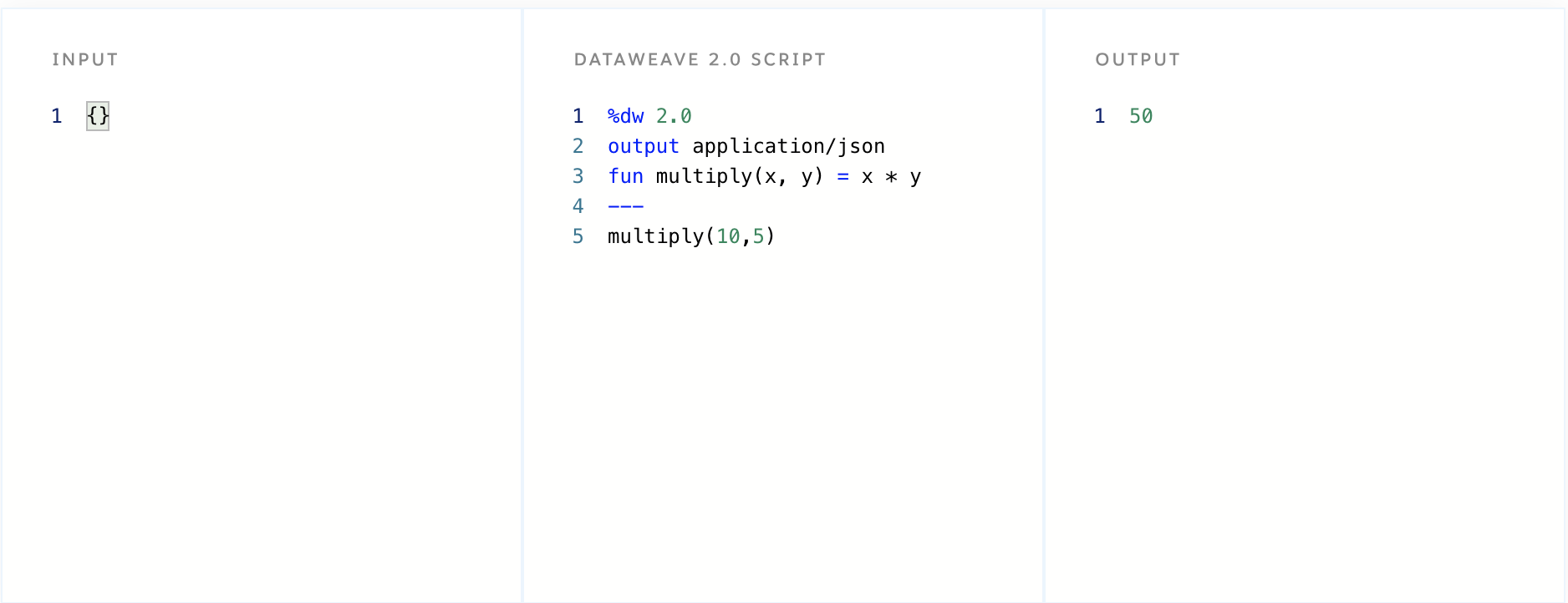Additionally, you can create functions that do one thing like multiply by 10. It accepts one parameter which is then multiplied by 10.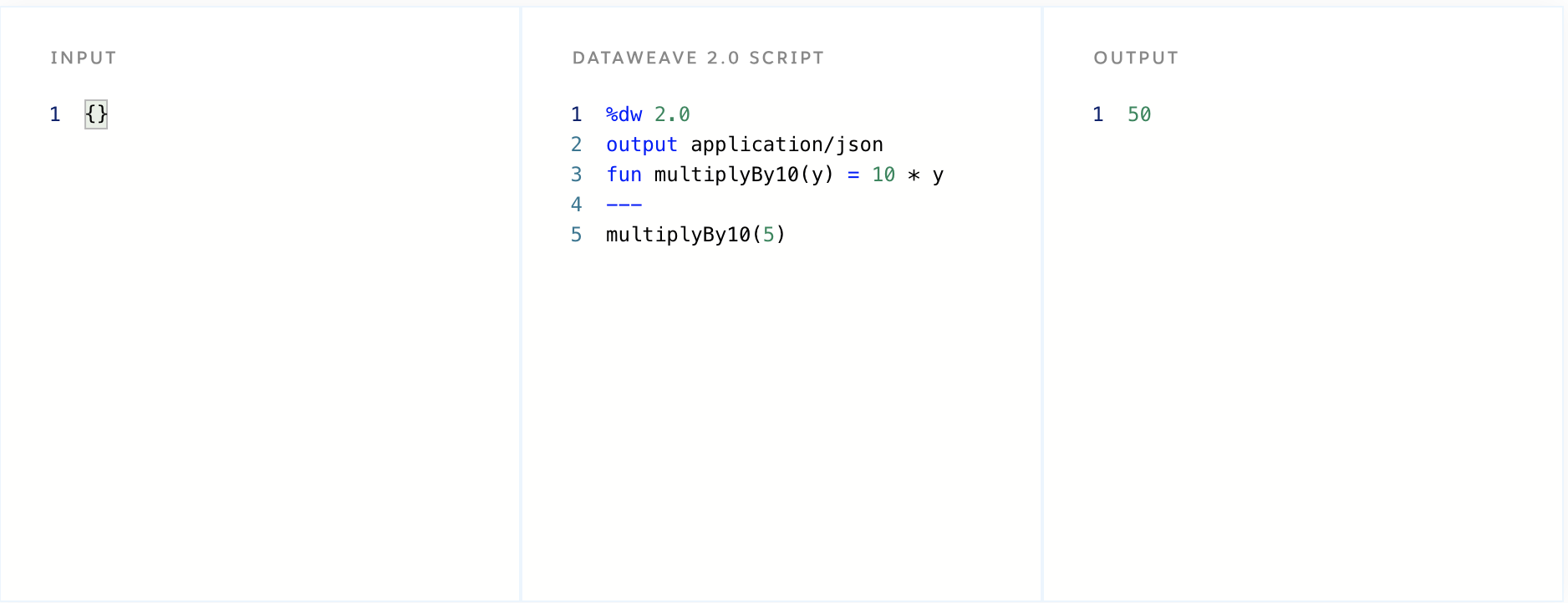## Currying a DataWeave function

In these examples, the parameters must be known and passed to the function at the time it is executed. But what if you don’t know what all the parameters are at the same time? Perhaps you only know the first parameter and don’t know when you will know the second parameter.

One way to resolve this would be to store the first parameter in a variable and wait until you know the second parameter before passing them to the functional and executing it: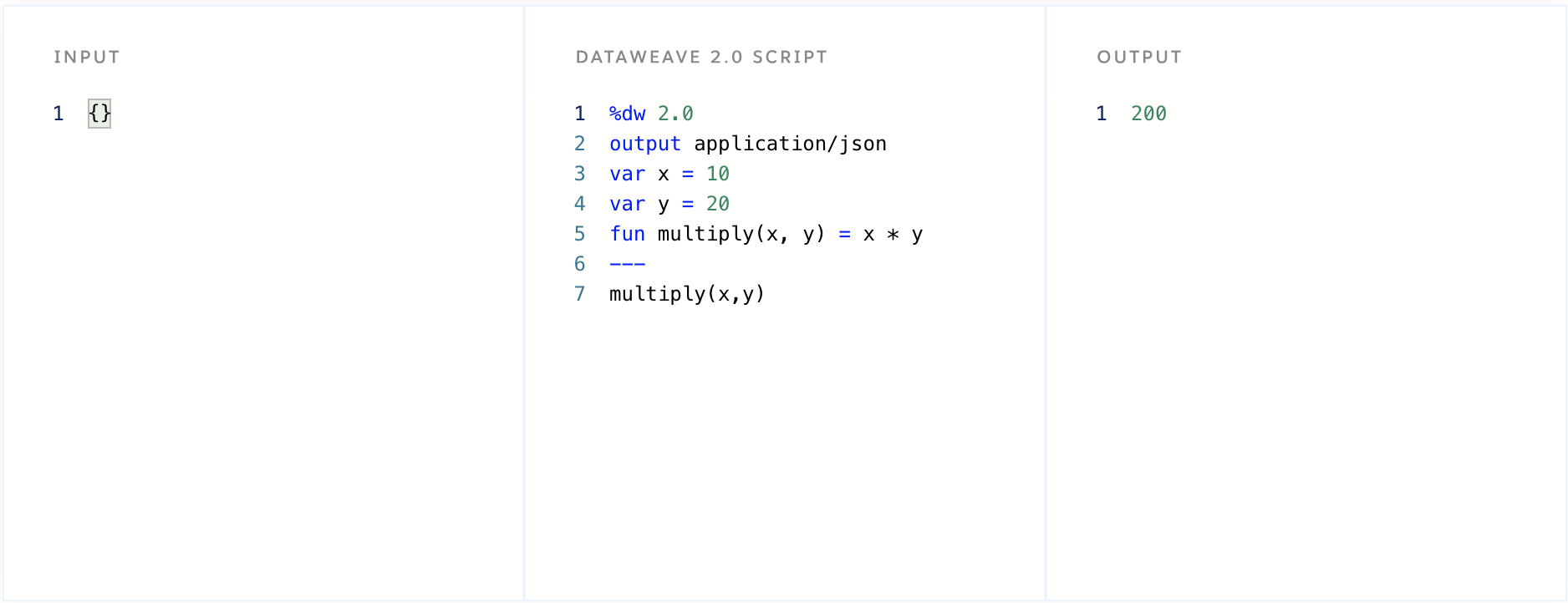However, it may be more convenient to fix the first parameter in the function and when the second parameter becomes known, fix and execute the function at that time. This is where currying can help. Take a look at the following DataWeave curried function: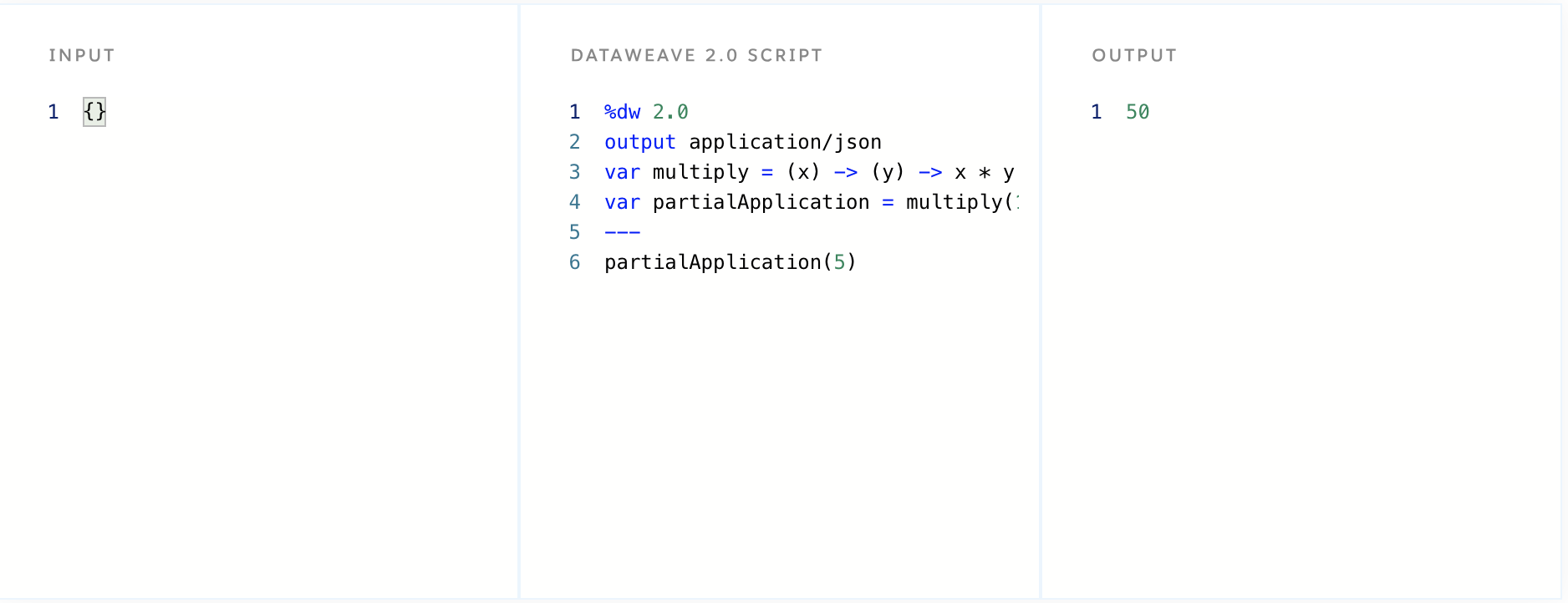It is functionally equivalent to the example discussed above (fun multiply(x, y) = x * y), however it will allow you to fix the x parameter before fixing the y parameter.

The x value has been fixed as 10 and the return value is another function that only expects one parameter, the y parameter. When the y parameter is passed the body of the function is executed, x and y are multiplied, and the result is returned, as shown in the example above, the y value is fixed as 5 and as x is already fixed as 10 the execution of the function returns the value 50.

This is referred to as the partial application of the function’s parameters as only some and not all parameters have been fixed, i.e. partially applied.

## Partial Application

What is a partial application? A partial application is a function that has had some of its parameters fixed inside its closure scope but not all. This is what we did above. The x parameter is fixed inside the function, resulting in a partially applied application (of the function).

The result of a partial application is a function that expects to receive the remaining unfixed parameter. Considering that DataWeave treats functions as first-class, it means that the resulting function of a partial application can be assigned to a variable and completed later. Consider the following example that requires four parameters. The curried function can be partially applied by passing only two parameters. The x and y parameters are fixed in the partially applied function twoDSpace.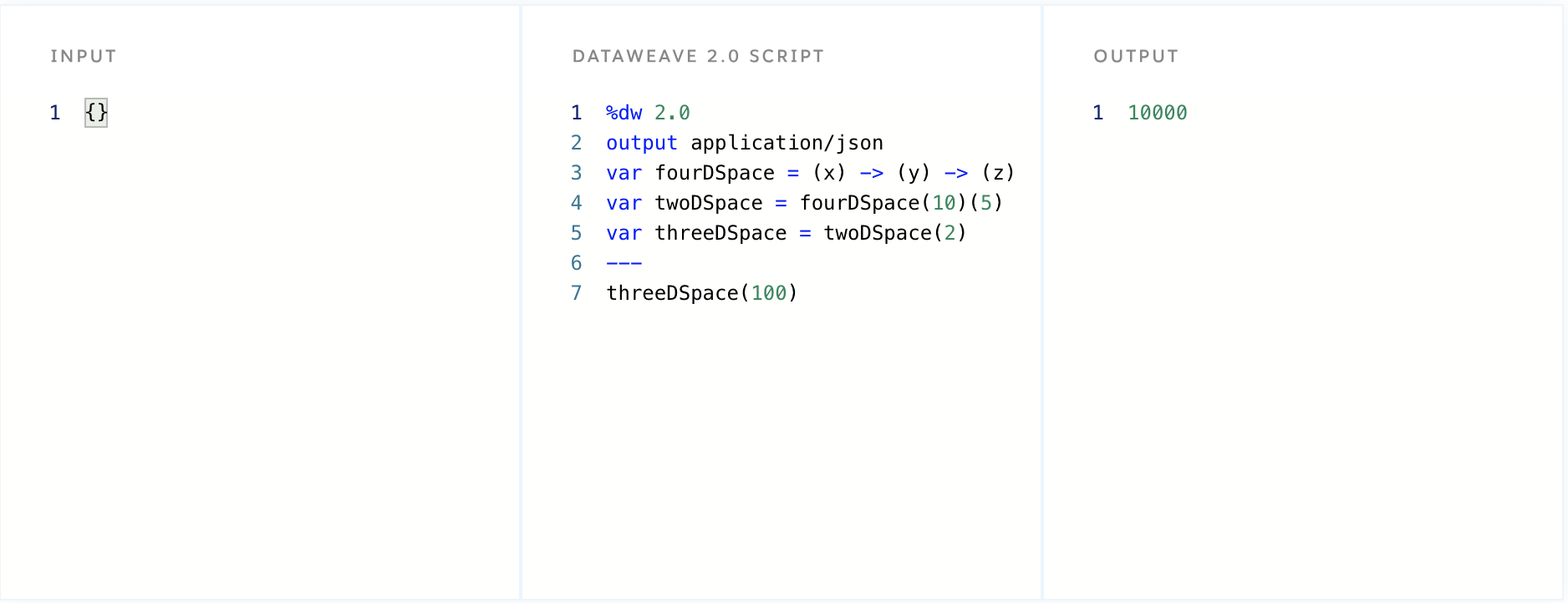Partial applications allow you to create specifications of a function. The following function creates a discount percentage, and the principle is discounted from each function. Each var below such as trousers, shoes, hat will reduce the number in the function by 10%. Check out a few examples below: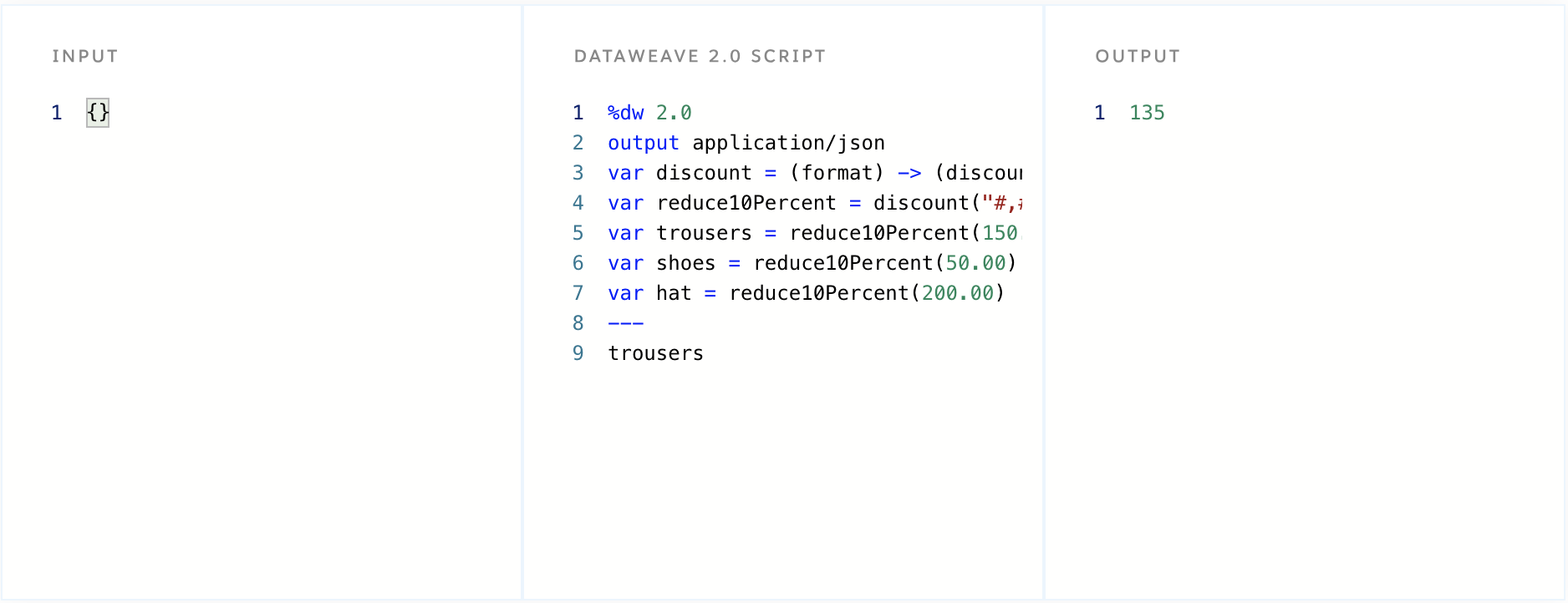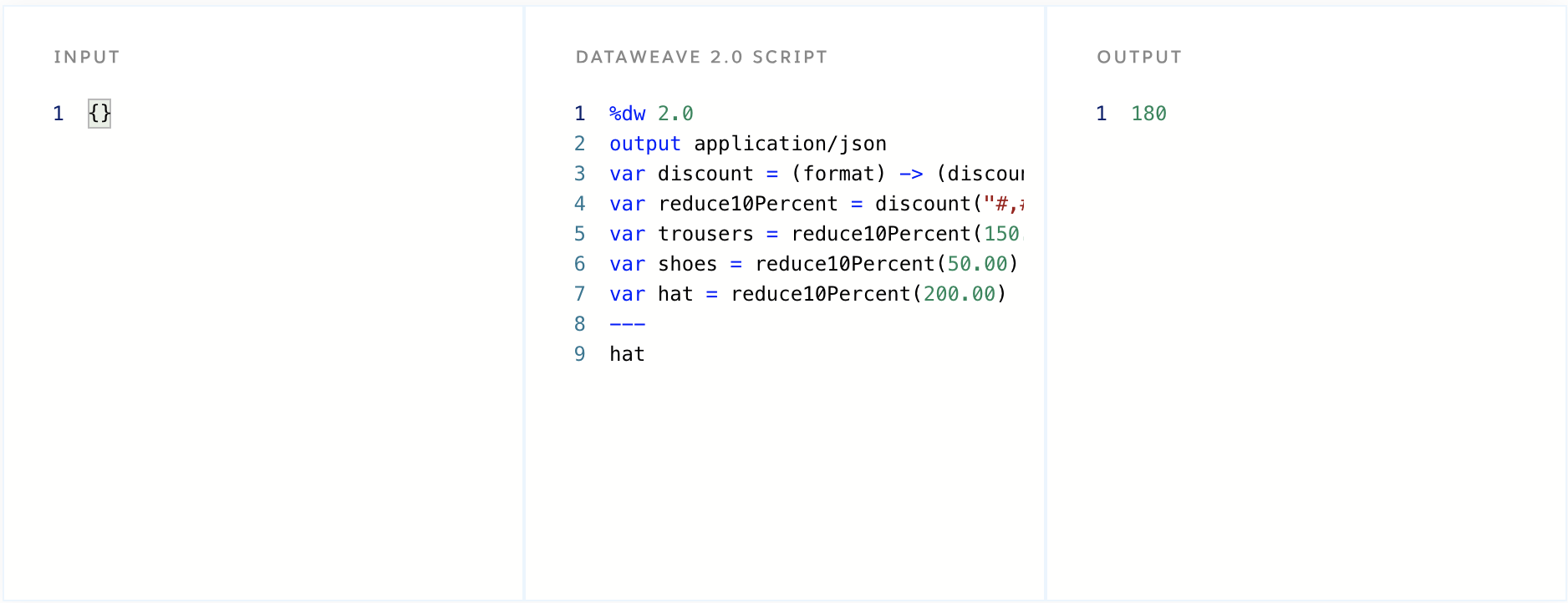## Functional composition and higher-order functions

Curried functions are useful in the context of functional composition. Referred to as higher-order functions, they are functions that accept another function as an argument and return another function or a result. Consider the following example which is composed using another function from these two functions: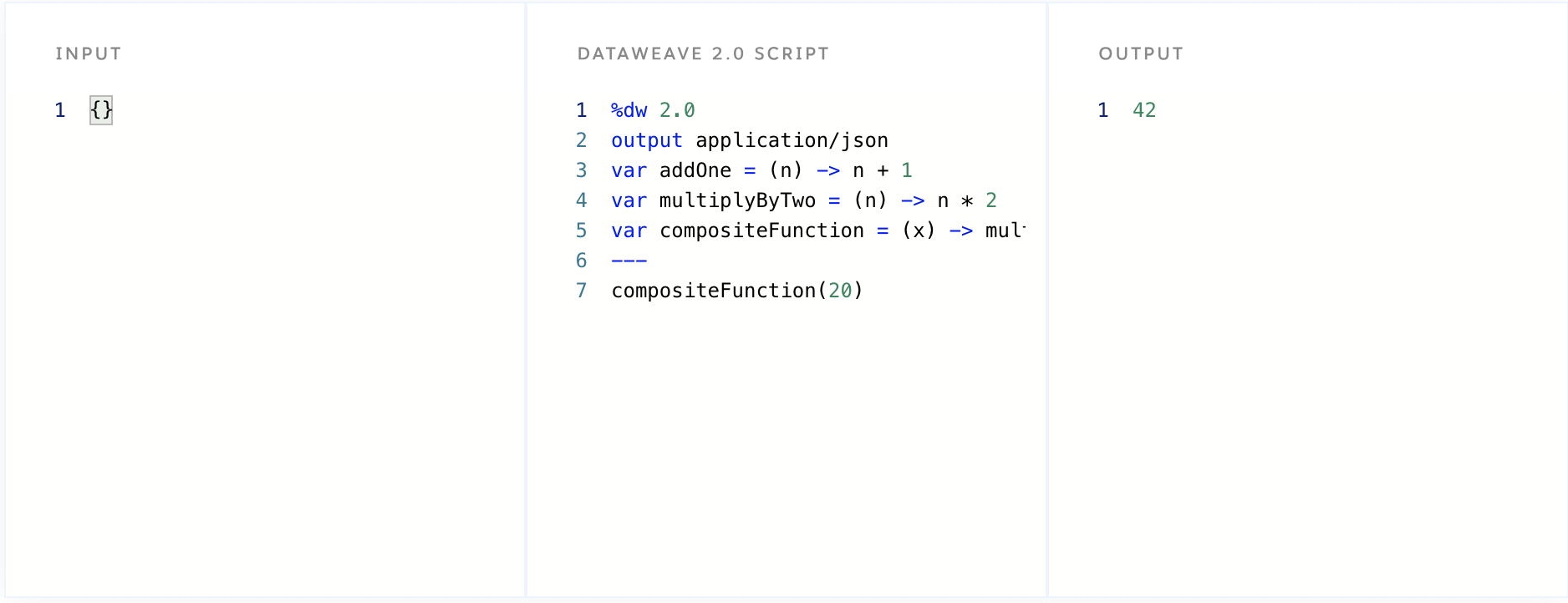## Partial application and functional composition

A more involved example is to partially apply a function that is then passed into another function and then the final parameter is fixed.

In this example, we are going to use the scrolling marquee HTML element that was so popular back in the day. In the code below, the direction of the scrolling marquee is fixed and the partial function is referenced with the openTag variable. The template compiles the elements that configure the scrolling marquee and include the opening and closing tags and the text to scroll. Additionally, the template function is fixed and the openTag is partially applied function is passed to the template function. It is then completed with the width function.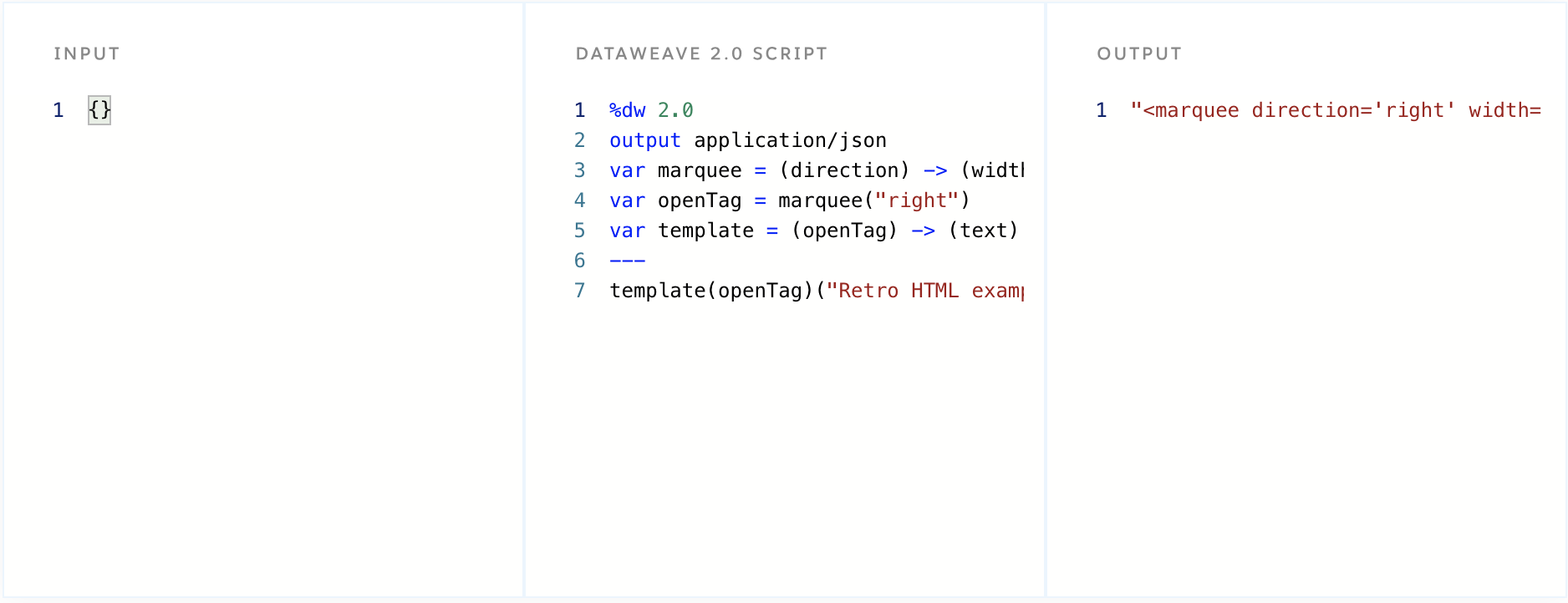## Conclusion

A curried function has many practical uses in the composition of reusable DataWeave scripts. With some study and plenty of practice, they can become a tool in the developer’s DataWeave toolbox. If you are interested in learning more about DataWeave, read our introductory series on What is DataWeave and also consider enrolling in the Anypoint Platform Development: DataWeave training course. Thank you so much for reading and please visit our developer tutorial home page for more tutorials.